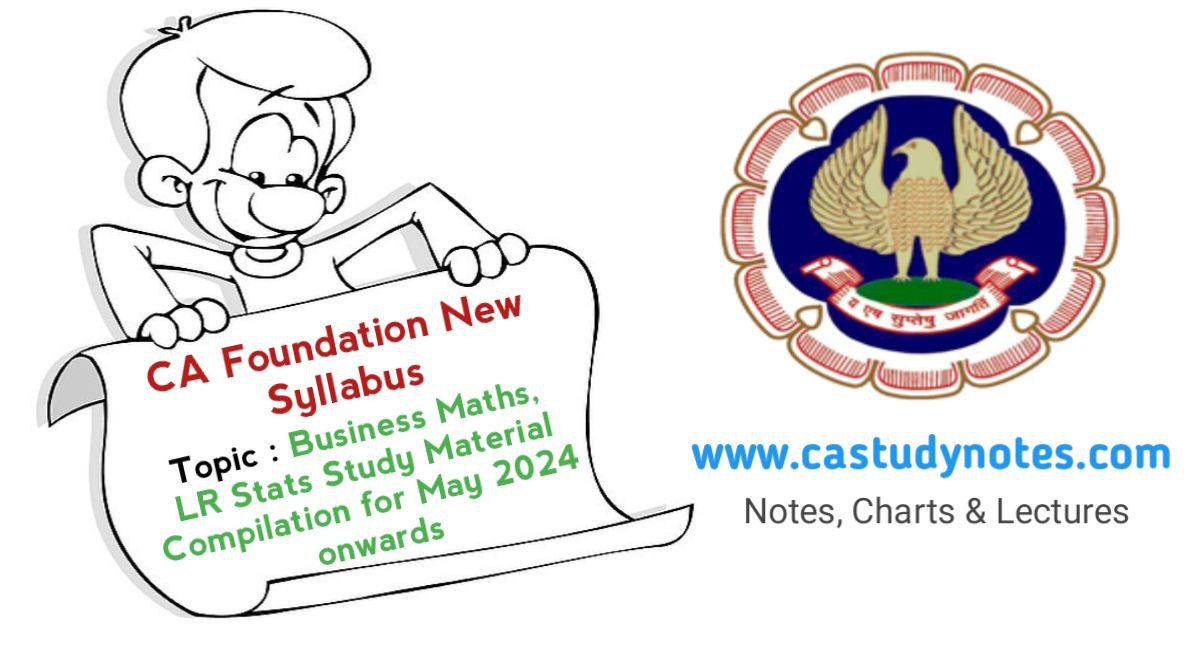# CA foundation Business Maths, LR & Statstic New Syllabus Study Material Compilation for May 2024 onwards

Hello Dear CA Students,

We are Sharing With You CA foundation Business Maths, LR & Statstic New Syllabus Study Material Compilation for May 2024 onwards Paper-3 : Business Maths, LR & Statstic . So kindly Check Out our http://www.castudynotes.com website and ALL the Best for Your upcoming Exams.

CA STUDY NOTES

# PAPER 3: QUANTITATIVE APTITUDE (100 MARKS)

### Objective

1. To develop an understanding of the basic mathematical and statistical tools and apply the same inbusiness, finance and economic situations.
2. To develop logical reasoning skills.

### Contents

The whole syllabus of Quantitative Aptitude shall be divided into three broad areas:

• Logical Reasoning
• Statistics

PART A: BUSINESS MATHEMATICS (40 MARKS)

1. Ratio and proportion, Indices and Logarithms: Ratio and proportion and Time and work-relatedproblems, Laws of Indices, Exponents and Logarithms and Anti Logarithms.
2. Equations: Equations: Linear Simultaneous linear equations up to three variables, Quadratic and Cubicequations in one variable. Applications in Business related problems.
3. Linear Inequalities: Linear Inequalities: Linear Inequalities in one and two variables and the solutionspace.

### Mathematics of Finance:

• Simple Interest
• Compound interest
• Nominal and Effective Rate of Interest
• Present Value
• Net Present Value
• Future Value
• Perpetuity
• Annuities
• Sinking Funds
• Calculating of EMI
• Calculations of Returns: Nominal and Effective rate of Return
• Compound Annual growth rate (CAGR)
1. Permutations and Combinations: Basic concepts of Permutations and combinations: Introduction, the factorial, permutations, results, circular permutations, permutations with restrictions, Combinations with standard results.
2. Sequence and Series: Introduction Sequences, Series, Arithmetic and Geometric progression, Relationship between AM and GM and Sum of n terms of special series and Business Applications.

### Sets, Relations, and Functions. Basics of Limits and Continuity functions.

1. Basic applications of Differential and Integral calculus in Business and Economics (Excluding thetrigonometric applications).

PART B: LOGICAL REASONING (20 MARKS)

1. Number series coding and Decoding and odd man out
2. Direction Tests
3. Seating Arrangements
4. Blood Relations

PART C: STATISTICS (40 MARKS)

1. Unit 1: Statistical Representation of Data: Diagrammatic representation of data, Frequency distribution, Graphical representation of Frequency Distribution – Histogram, Frequency Polygon, Ogive,Pie-chart.

Unit:2 Sampling: Basic principles of sampling theory, comparison between sample survey and complete enumeration, some important terms associated sampling types of sampling, sampling andnon-sampling errors.

Measures of Central tendency and Dispersion: Measures of Central Tendency and Dispersion: Mean Median, Mode, Mean Deviation, Quartiles and Quartile Deviation, Standard Deviation, Co-efficient of Variation, Coefficient of Quartile Deviation.

Probability: Independent and dependent events; mutually exclusive events. Total and CompoundProbability and Bayes’ theorem.

Theoretical Distributions: Random variables, Discrete and Continuous Random variables, Expectation of a discrete random variable, Theoretical Distributions: Binomial Distribution, Poisson distribution – basic application and Normal Distribution – basic applications.

Correlation and Regression: Scatter diagram, Karl Pearson’s Coefficient of Correlation Rank Correlation, Regression lines, Regression equations, Regression coefficients.

Index Numbers: Uses of Index Numbers, Problems involved in construction of Index Numbers, Methodsof construction of Index Numbers. BSE SENSEX and NSE.